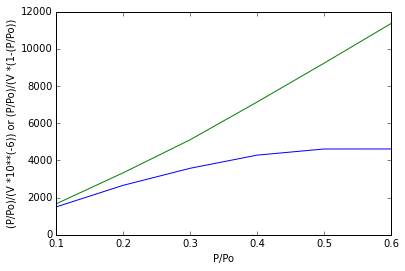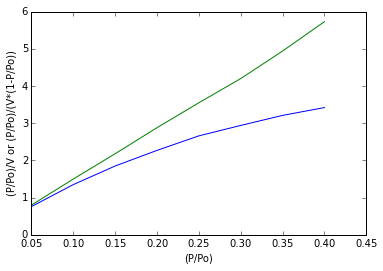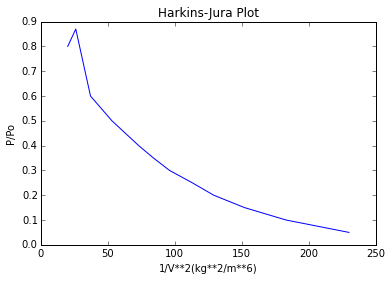## Page 986 Example 17.1¶

In :
%matplotlib inline
from matplotlib.pyplot import plot,title,show,xlabel,ylabel

#For 1 m3 of pellet with a voidage ε, then
#Number of particles = (1 − ε)/(π/6)(15 × 10**−9)**3
#Surface area per unit volume = (1 − ε)π(15 × 10−9)2/(π/6)(15 × 10**−9)**3
#= 6(1 − ε)/(15 × 10**(−9)) m**2/m**3
#1 m**3 of pellet contains 2290(1- ε)kg solid and hence:
specific_surface = 6/(15*10**(-9)*2290)
print"\n Specific surface = %.3f*10**5 m**2/kg "%(specific_surface*10**(-5))
#(a) Using the BET isotherm

#y = (P/Po)/V *10**(-6)
y = [1500, 2660, 3576 ,4283 ,4613, 4615]
#y1 = (P/Po)/V *(1-(P/Po)
y1 = [1666 ,3333 ,5109, 7138, 9226, 11358]
#x = P/Po
x = [0.1 ,0.2 ,0.3 ,0.4 ,0.5 ,0.6]
plot(x,y)
xlabel("P/Po")
ylabel("(P/Po)/(V *10**(-6)) or (P/Po)/(V *(1-(P/Po))")
plot(x,y1)
show()

#intercept, 1/VB = 300, and slope, (B − 1)/VB = 13,902
B = (13902/300)+1
print"\n B = %.2f "%(B)
V = 1/(300*47.34)#
#Total surface area
S = ((70.4*10**(-6)*808*6.2*10**(26)*0.162*10**(-18)))/28
print"\n Total surface area = %.3f *10**5 m**2/kg"%(S*10**(-5))

#(b) Using the Langmuir form of the isotherm

#Slope 1/V = 13,902
V = 1/13902
print"\n V = %.1f*10**(-6)m**3/kg"%(V*10**6)
#which agrees with the value of the BET isotherm
#It may be noted that areas calculated from the isotherm are some 20 per cent greater than the geometric surface, probably due to the existence of some internal surface within the particles

 Specific surface = 1.747*10**5 m**2/kgB = 47.00

Total surface area = 2.040 *10**5 m**2/kg

V = 0.0*10**(-6)m**3/kg


## Page 1000 Example 17.2¶

In :
%matplotlib inline
from matplotlib.pyplot import plot,title,show,xlabel,ylabel

#(a)
#For n = 1,
#(P/P0)/V= (P/P0)V1+ 1/B2V1
#where V is the gas phase volume equivalent to the amount adsorbed
#x = (P/Po)
x = [0.05, 0.10 ,0.15 ,0.20 ,0.25 ,0.30, 0.35, 0.40]
#y = (P/Po)/V in kg/m**3
y = [0.76 ,1.35, 1.85, 2.27, 2.66, 2.94 ,3.21, 3.42]
#y1 = (P/Po)/(V*(1-P/Po)) in kg/m**3
y1 = [0.80, 1.50, 2.18 ,2.88, 3.55, 4.20 ,4.94, 5.73]
plot(x,y)
plot(x,y1)
xlabel("(P/Po)")
ylabel("(P/Po)/V or (P/Po)/(V*(1-P/Po))")
show()

#The data, which are plotted as (P /P 0)/V against P/P0  may be seen    to conform to a straight line only at low values of P/P0, suggestin    g that more than one layer of molecules isadsorbed.
Slope = 12.56
V1 = 1/12.56
#The surface area occupied by this absorbed volume
S = V1*6.02*10**(26)*0.162*10**(-18)/24
print"\n S = %d m**2/kg"%(S)

#(b)
#P/P0/V*(1 − P/P0)= 1/V1*B2+(B2−1)/(V1*B2)*(P /P 0)
#y2 = (P/Po)
#x2 = 1V**2
y2 = [0.05, 0.10, 0.15, 0.20, 0.25, 0.30 ,0.35, 0.40, 0.50 ,0.60 ,0.87, 0.80]
x2 = [230 ,183 ,152 ,129, 113, 96, 84 ,73 ,53 ,37 ,26 ,20]

plot(x2,y2)
title("Harkins-Jura Plot")
xlabel("1/V**2(kg**2/m**6)")
ylabel("P/Po")
show()S = 323527 m**2/kg## Page 1015 Example 17.3¶

In :
from math import pi

#For case (a):
#Cs = 10C which represents a linear isotherm.
#All concentrations move at the same velocity.If z0=0 at t = 0 for a    ll concentrations, the adsorption wave propagates as a step change     from the inlet to the outlet concentration.
#f (Cs ) = 10
#u = 1×10**(−4)/[(π/4)*(0.15**(2)*ε)] m/s
#where ε is the intergranular voidage = 0.4
e = 0.4
m = e/(1-e)
print"\n m=%f"%(m)
t = 3600#              #time is in secs
z = ((4*10**(-4))/(pi*(0.15**2)*0.4))*(3600/(1+10*(0.6/0.4)))
print"\n z = %.2f m"%(z)

#It may be noted that, when the adsorption wave begins to emerge from the bed, the bed is saturated in equilibrium with the inlet concentration.
#Hence: uAεtC0 = zA[εC0 + (1 − ε)Cs ]

#For case (b):
#Cs = 3C0.3 which represents a favourable isotherm.
#As C increases, f(C) decreases and points of higher concentrations are predicted to move a greater distance in a given time than lower concentrations. It is not possible for points of higher concentrationsto overtake lower concentrations, and if z0=0 for all concentrations,the adsorption wave will propagate as a step change similar to case a.

#Hence: z = ut/[1+(1/m)(Cs/Co)]
Co = 0.003#              #in kmol/m**3
z = ((4*10**(-4)*5/((pi)*0.4*0.15**2)))*(3600/(1+(0.6/0.4)*(3/Co**0.7)))
print"\n z = %.2f m"%(z)

#For case (c):
#Cs = (10**(4))*(C**2) which represents an unfavourable isotherm.
#f(C)=2* 10**4* C.
#As C increases, f (C) increases such that, in a given time, z for lower concentrations is greater than for higher concentrations. Following the progress of the breakpoint concentration,C = 0.003 kmol/m**3,then:
#f(0.003) = 60
z1 = (4*10**(-4)/(pi*0.15**(2)*0.4))*(3600/(1+(0.60/0.40)*60))
print"\n For case (c)"
print"\n z = %.2f m"%(z1)

#At breakpoint,the bed is far from saturated and:
saturation = 100*((4*10**(-4)*3600)/(pi*(0.15**(2)*0.4)))/(0.55*(1+(0.6/0.4)*(9/0.03)))
print"\n saturation = %.1f per cent"%(saturation)

 m=0.666667

z = 3.18 m

z = 0.97 m

For case (c)

z = 0.56 m

saturation = 20.5 per cent


## Page 1031 Example 17.5¶

In :
from sympy import symbols,solve

#let x = [f(C)]mean
x = (8.4*10**(-2)*1266)/(2.67*10**(-3)*1.186)

#e is porosity
e = 0.47
m = e/(1-e)
print"\n m = %.2f"%(m)

#The velocity uc with which the adsorption wave moves through the column may be obtained from equation 17.79
uc = 6.2*10**(-6)
density = 1266#               #density is in kg/m**3
Gs = uc*(1-e)*density
print"\n Gs = %.2f*10**(-3) kg/m**2.sec "%(Gs*10**3)

#From overall balance:
#We have given eq: Gs1(0.084-0)=0.129(0.00267)
#On solving above eq :
Gs1 = symbols('Gs1')
Gs2 = solve(Gs1*(0.084-0)-0.129*(0.00267))
print"\n Gs1 = %.2f*10**(-3) kg/m**2.sec"%(Gs2*1000)

#Part(b)
#time ta for the adsorption zone to move its own length za is given by:
za = 0.185
ta =(za/uc)/3600
print"\n ta = %.1f hrs"%(ta)#

#The time taken for a point at a distance z into the zone to emerge is given by:
#t = (z1/za)ta
yr = [0.0001, 0.0002, 0.0006, 0.0010 ,0.0014 ,0.0018, 0.0022, 0.0024]
yre = [0.00005, 0.0001, 0.00032, 0.00062, 0.00100, 0.00133, 0.00204, 0.00230]
I=0
I1 = 0
i=0
print"\nI= %.1f"%(I)
Y=[]
while i <=7:
y.append(1/(yr[(i)]-yre[(i)]))
if i>0:
I = I + (yr[(i)]-yr[(i-1)])*(y[(i)]+y[(i-1)])/2
print"\n I=%f"%(I)

i=i+1#

z_za = [0, 0.137, 0.362, 0.473, 0.560 ,0.674, 0.852, 1.000]
t=[]
for zza in z_za:t.append((zza)*ta)
j=0
while j<=7:
print"\ntime = %.1f h"%(t[(j)])
j=j+1

 m = 0.89

Gs = 4.16*10**(-3) kg/m**2.sec

Gs1 = 4.10*10**(-3) kg/m**2.sec

ta = 8.3 hrs

I= 0.0

I=0.000106

I=0.000745

I=0.001570

I=0.002556

I=0.003675

I=0.004906

I=0.005568

time = 0.0 h

time = 1.1 h

time = 3.0 h

time = 3.9 h

time = 4.6 h

time = 5.6 h

time = 7.1 h

time = 8.3 h